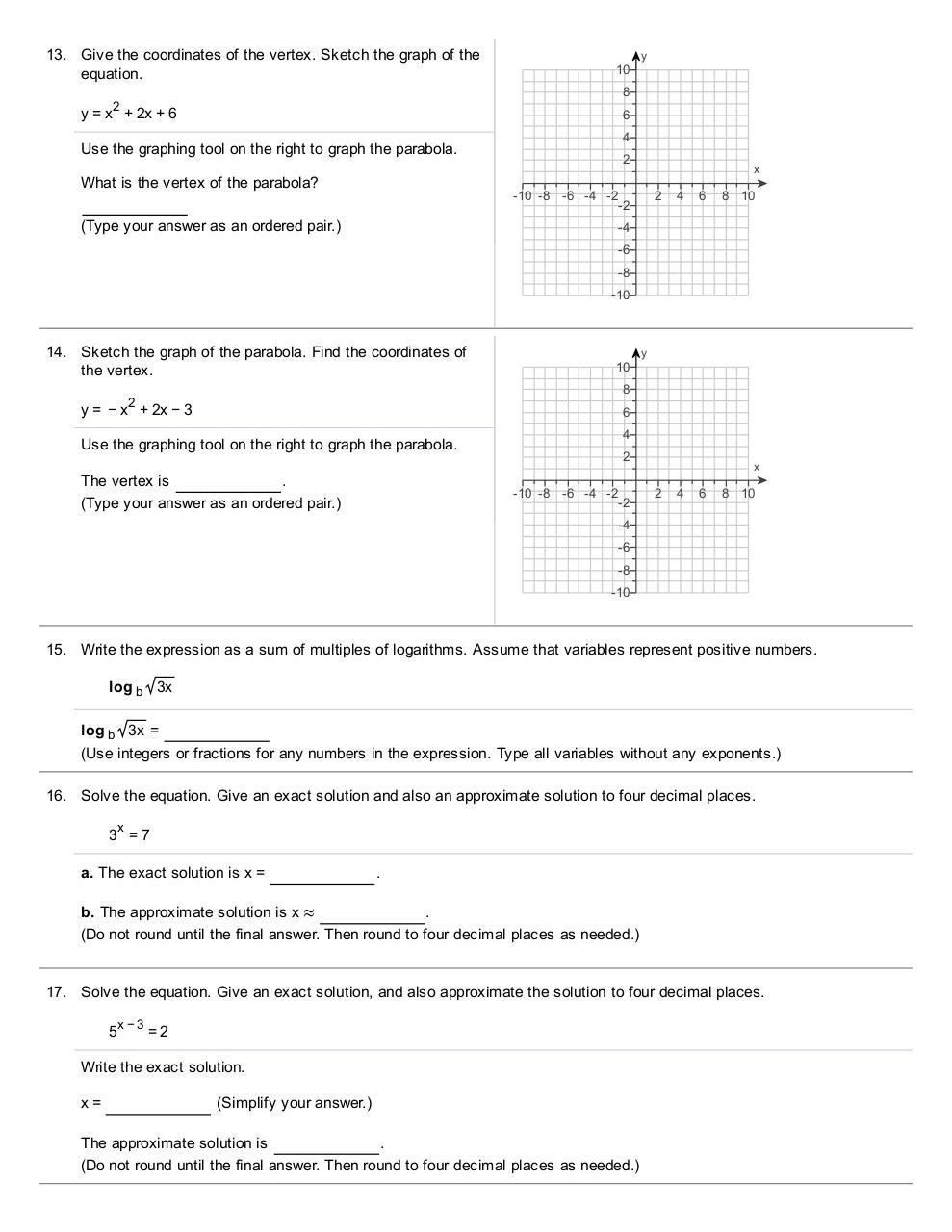# College Level.pdfPage 1 2 3 4 5 6 7 8 9 10

#### Text preview

13. Give the coordinates of the vertex. Sketch the graph of the
equation.

10

y

8

2

y = x + 2x + 6

6

Use the graphing tool on the right to graph the parabola.
What is the vertex of the parabola?

4
2

x

­10 ­8 ­6 ­4 ­2
­2

(Type your answer as an ordered  pair.)

2

4

6

8 10

­4
­6
­8
­10

14. Sketch the graph of the parabola. Find the coordinates of
the vertex.

10

y

8

y = − x 2 + 2x − 3

6

Use the graphing tool on the right to graph the parabola.
The vertex is

(Type your answer as an ordered  pair.)

4
2
­10 ­8 ­6 ­4 ­2
­2

x

2

4

6

8 10

­4
­6
­8
­10

15. Write the expression as a sum of multiples of logarithms. Assume that variables represent positive numbers.
log b 3x
log b 3x =
(Use integers or fractions for any numbers in the expression. Type all variables without any  exponents.)
16. Solve the equation. Give an exact solution and also an approximate solution to four decimal places.
x

3 =7
a. The exact solution is x =

.

b. The approximate solution is x ≈
.
(Do not round until the final answer. Then round to four decimal places as  needed.)

17. Solve the equation. Give an exact solution, and also approximate the solution to four decimal places.
x −3

5

=2

Write the exact solution.
x=